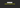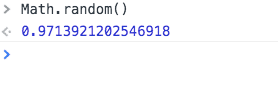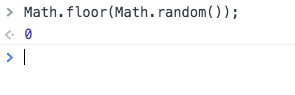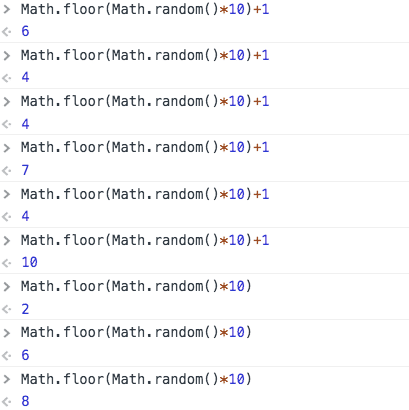# How to generate random numbers in JavaScriptIn this tutorial, we are going to learn how to generate random numbers in javascript by using `Math.random()` method.

By default `Math.random()` method generates a floating point number between 0 to 1 (inclusive of 0, but not 1).

``Math.random();``We don’t need floating point numbers so that by using `Math.floor( )` method we floor it down to a decimal number.

``Math.floor(Math.random());``### Let’s generate random numbers ranging between 1- 10.

``Math.floor(Math.random()*10) //random numbers 0-9``

The above code only generates random numbers between 0 –9. But we need random numbers between 1–10.

``Math.floor(Math.random()*10)+1; //random numbers 1-10``### 1–100 random numbers

``Math.floor(Math.random()*100)+1; //random numbers from 1-100``

Happy coding…

## Top Udemy Courses##### JavaScript - The Complete Guide 2020 (Beginner + Advanced)
45,614 students enrolled
52 hours of video content
View Course##### React - The Complete Guide (incl Hooks, React Router, Redux)
284,472 students enrolled
40 hours of video content
View Course##### Vue JS 2 - The Complete Guide (incl. Vue Router & Vuex)
130,921 students enrolled
21 hours of video content
View Course